Q

# Try this! The logic circuit shown below has the input waveforms 'A' and 'B' as shown. Pick out the correct output waveform.Output is

The logic circuit shown below has the input waveforms 'A' and 'B' as shown. Pick out the correct output waveform.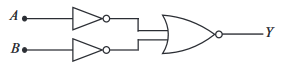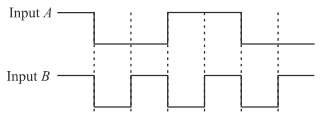Output is

• Option 1)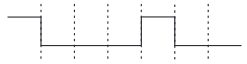• Option 2)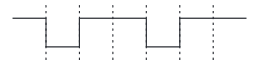• Option 3)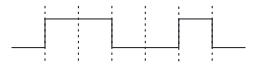• Option 4)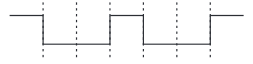891 Views

As we learnt in

NOT gate -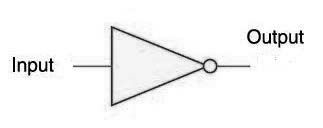- wherein

A is  input

Y is out put

NOR Gate -

NOT + OR  Gate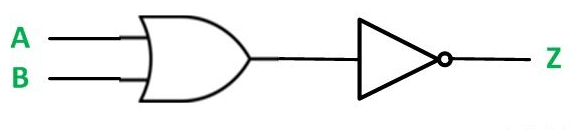- wherein

A and B are input

Y is out put

Truth table for the given circuit is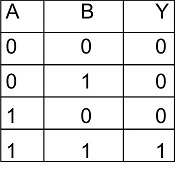Correct option is 1.

Option 1)This is the correct option.

Option 2)This is an incorrect option.

Option 3)This is an incorrect option.

Option 4)This is an incorrect option.

Exams
Articles
Questions